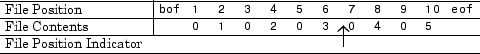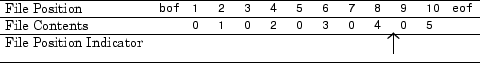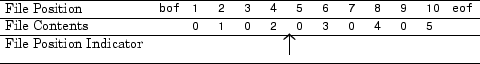Main Content

## Import Binary Data with Low-Level I/O

### Low-Level Functions for Importing Data

Low-level file I/O functions allow the most direct control over reading or writing data to a file. However, these functions require that you specify more detailed information about your file than the easier-to-use high-level functions. For a complete list of high-level functions and the file formats they support, see Supported File Formats for Import and Export.

If the high-level functions cannot import your data, use one of the following:

• `fscanf`, which reads formatted data in a text or ASCII file; that is, a file you can view in a text editor. For more information, see Reading Data in a Formatted Pattern.

• `fgetl` and `fgets`, which read one line of a file at a time, where a newline character separates each line. For more information, see Reading Data Line-by-Line.

• `fread`, which reads a stream of data at the byte or bit level. For more information, see Reading Binary Data in a File.

Note

The low-level file I/O functions are based on functions in the ANSI® Standard C Library. However, MATLAB® includes vectorized versions of the functions, to read and write data in an array with minimal control loops.

### Reading Binary Data in a File

As with any of the low-level I/O functions, before importing, open the file with `fopen`, and obtain a file identifier. When you finish processing a file, close it with `fclose``(fileID)`.

By default, `fread` reads a file 1 byte at a time, and interprets each byte as an 8-bit unsigned integer (`uint8`). `fread` creates a column vector, with one element for each byte in the file. The values in the column vector are of class `double`.

For example, consider the file `nine.bin`, created as follows:

```fid = fopen('nine.bin','w'); fwrite(fid, [1:9]); fclose(fid);```

To read all data in the file into a 9-by-1 column vector of class `double`:

```fid = fopen('nine.bin'); col9 = fread(fid); fclose(fid);```

#### Changing the Dimensions of the Array

By default, `fread` reads all values in the file into a column vector. However, you can specify the number of values to read, or describe a two-dimensional output matrix.

For example, to read `nine.bin`, described in the previous example:

```fid = fopen('nine.bin'); % Read only the first six values col6 = fread(fid, 6); % Return to the beginning of the file frewind(fid); % Read first four values into a 2-by-2 matrix frewind(fid); two_dim4 = fread(fid, [2, 2]); % Read into a matrix with 3 rows and % unspecified number of columns frewind(fid); two_dim9 = fread(fid, [3, inf]); % Close the file fclose(fid);```

#### Describing the Input Values

If the values in your file are not 8-bit unsigned integers, specify the size of the values.

For example, consider the file `fpoint.bin`, created with double-precision values as follows:

```myvals = [pi, 42, 1/3]; fid = fopen('fpoint.bin','w'); fwrite(fid, myvals, 'double'); fclose(fid);```

To read the file:

```fid = fopen('fpoint.bin'); % read, and transpose so samevals = myvals samevals = fread(fid, 'double')'; fclose(fid);```

For a complete list of precision descriptions, see the `fread` function reference page.

#### Saving Memory

By default, `fread` creates an array of class `double`. Storing double-precision values in an array requires more memory than storing characters, integers, or single-precision values.

To reduce the amount of memory required to store your data, specify the class of the array using one of the following methods:

• Match the class of the input values with an asterisk (`'*'`). For example, to read single-precision values into an array of class `single`, use the command:

`mydata = fread(fid,'*single')`
• Map the input values to a new class with the `'=>'` symbol. For example, to read `uint8` values into an `uint16` array, use the command:

`mydata = fread(fid,'uint8=>uint16')`

For a complete list of precision descriptions, see the `fread` function reference page.

### Reading Portions of a File

MATLAB low-level functions include several options for reading portions of binary data in a file:

#### Testing for End of File

When you open a file, MATLAB creates a pointer to indicate the current position within the file.

Note

Opening an empty file does not move the file position indicator to the end of the file. Read operations, and the `fseek` and `frewind` functions, move the file position indicator.

Use the `feof` function to check whether you have reached the end of a file. `feof` returns a value of `1` when the file pointer is at the end of the file. Otherwise, it returns `0`.

For example, read a large file in parts:

```filename = 'largedata.dat'; % hypothetical file segsize = 10000; fid = fopen(filename); while ~feof(fid) currData = fread(fid, segsize); if ~isempty(currData) disp('Current Data:'); disp(currData); end end fclose(fid);```

#### Moving within a File

To read or write selected portions of data, move the file position indicator to any location in the file. For example, call `fseek` with the syntax

`fseek(fid,offset,origin);`

where:

• `fid` is the file identifier obtained from `fopen`.

• `offset` is a positive or negative offset value, specified in bytes.

• `origin` specifies the location from which to calculate the position:

 `'bof'` Beginning of file `'cof'` Current position in file `'eof'` End of file

Alternatively, to move easily to the beginning of a file:

`frewind(fid);`

Use `ftell` to find the current position within a given file. `ftell` returns the number of bytes from the beginning of the file.

For example, create a file `five.bin`:

```A = 1:5; fid = fopen('five.bin','w'); fwrite(fid, A,'short'); fclose(fid);```

Because the call to `fwrite` specifies the `short` format, each element of `A` uses two storage bytes in `five.bin`.

Reopen `five.bin` for reading:

`fid = fopen('five.bin','r');`

Move the file position indicator forward 6 bytes from the beginning of the file:

```status = fseek(fid,6,'bof'); ```Read the next element:

`four = fread(fid,1,'short');`

The act of reading advances the file position indicator. To determine the current file position indicator, call `ftell:`

```position = ftell(fid) position = 8 ```To move the file position indicator back 4 bytes, call `fseek` again:

`status = fseek(fid,-4,'cof');`Read the next value:

`three = fread(fid,1,'short');`

### Reading Files Created on Other Systems

Different operating systems store information differently at the byte or bit level:

• Big-endian systems store bytes starting with the largest address in memory (that is, they start with the big end).

• Little-endian systems store bytes starting with the smallest address (the little end).

Windows® systems use little-endian byte ordering, and UNIX® systems use big-endian byte ordering.

To read a file created on an opposite-endian system, specify the byte ordering used to create the file. You can specify the ordering in the call to open the file, or in the call to read the file.

For example, consider a file with double-precision values named `little.bin`, created on a little-endian system. To read this file on a big-endian system, use one (or both) of the following commands:

• Open the file with

`fid = fopen('little.bin', 'r', 'l')`
• Read the file with

`mydata = fread(fid, 'double', 'l')`

where `'l'` indicates little-endian ordering.

If you are not sure which byte ordering your system uses, call the `computer` function:

`[cinfo, maxsize, ordering] = computer`
The returned `ordering` is `'L'` for little-endian systems, or `'B'` for big-endian systems.

Download ebook